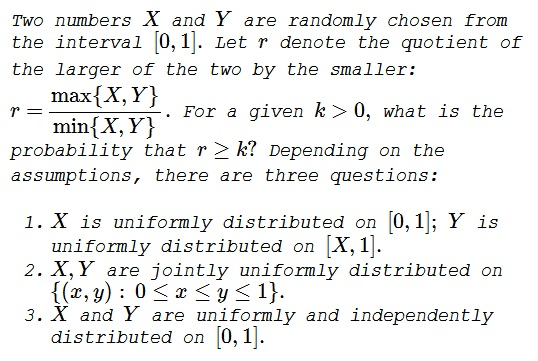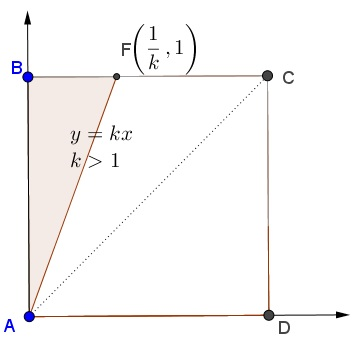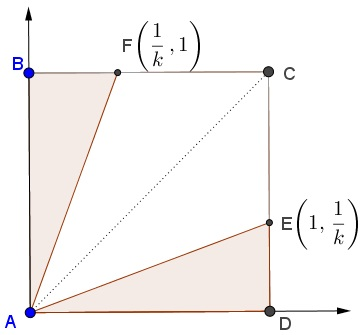Quotient Estimates

ProblemQuestion 1, Solution 1

Suppose $X$ is uniformly randomly chosen in $[0,1]$ and $Y$ is uniformly randomly chosen in $[X,1]$. The ratio $\displaystyle r=\frac{Y}{X}$ is a random variable with the CDF:

$P(r\geq k)=P(Y\geq kX)=\int P(Y\geq kX|X=x)dP(X=x)$

Since $Y$ is drawn from $[X,1]$, $\displaystyle P(Y\geq kX|X=x)=1-\frac{x}{1-x}(k-1)$ for $\displaystyle 1\leq k\leq \frac{1}{x}$ and 0 for $\displaystyle k> \frac{1}{x}$.

Therefore, for a fixed $k\geq 1$, the interval of the integration above is $\displaystyle 0\leq x\leq \frac{1}{k}$, and $dP(X=x)=dx$.

\displaystyle\begin{align} P(r\geq k)=P(Y\geq kX)&=\int_0^{\frac{1}{k}} 1-\frac{x}{1-x}(k-1) dx\\ &=1-(k-1)\ln\frac{k-1}{k}\end{align}

Question 1, Solution 2

Given $x$,

\displaystyle\begin{align}P(Y\gt kX)&=P(y\in (kx,1))=\int_{kx}^1\frac{1}{1-x} dy\\ &=\frac{1-kx}{1-x}, 0 \lt x \lt \frac{1}{k} \end{align}

Hence, in the general case,

\displaystyle \begin{align}P(Y\gt kX)&=\int_0^{1/k}\frac{1-kx}{1-x}dx\\ &=1+(1-k)\ln(1-1/k). \end{align}

Question 1, Solution 3

We can reduce this case to the distribution of

$\displaystyle \omega:=\max\left\{\frac{X}{(1-X) Y+X},\frac{(1-X) Y+X}{X}\right\}=\frac{(1-X) Y+X}{X}$

where both variables are uniform in $[0,1]$, with

$\displaystyle f_\omega(\omega)=\log \left(\frac{\omega }{\omega -1}\right)-\frac{1}{\omega }$

Then

$\displaystyle \mathbb{P}(r>k)=\int_k^{\infty } \log \left(\frac{\omega }{\omega -1}\right)-\frac{1}{\omega }\, d\omega=(1-k) (\log (k-1)-\log (k))$

Question 2, Solution 1

With the reference to the diagram below, $\{(x,y):\, 0\le x\le y\le 1\}=\Delta ABC.$In $\Delta ABC,$ $y\ge x,$ hence, always $r\ge 1.$ It follows that, for $k\le 1,$ the probability $P(r\ge k)=1.$

For the case $k\ge 1,$ having a joint uniform distribution means that the probability of $(X,Y)$ falling into a given subset of $\Delta ABC$ is proportional to the area of the subset. Now, for a fixed $k\ge 1,$ $r\ge k$ in $\Delta ABF,$ where $\displaystyle F=\left(\frac{1}{k},1\right).$ It follows that $\displaystyle P(r\ge k)=\frac{[\Delta ABF]}{[\Delta ABC]}=\frac{1}{k}.$ (Here $[F]$ denotes the area of shape $F.)$

Question 3, Solution 1

$X$ and $Y$ being uniformly distributed on $[0,1]$ means that their probability densities are defined by $f_X(x)=1,$ $x\in [0,1]$ and $0$ elsewhere. Similarly, $f_Y(y)=1,$ $y\in [0,1]$ and $0$ elsewhere. The two being independent, their joint density is the product of the individual two: $f_{X,Y}(x,y)=f_X(x)f_Y(y).$ It follows that $f_{X,Y}(x,y)=1$ for $(x,y)\in[0,1]\times [0,1]$ and $0$ elsewhere. In other words, the joint distribution is uniform, and the reasoning used to answer Question 1 applies here as well.

With the reference to the diagram below, for a given $k\ge 1,$ $\displaystyle \frac{Y}{X}\ge k$ in $\Delta ABF$ and $\displaystyle \frac{X}{Y}\ge k$ in $\Delta ADE.$It follows that $\displaystyle \{(x,y):\, \frac{\max\{X,Y\}}{\min\{X,Y\}}\}=\Delta ABF\cup\Delta ADE.$ As a consequence, for $k\ge 1,$

$\displaystyle P(r\ge k)=\frac{[\Delta ABF]+[\Delta ADE]}{\Delta ABCD]}=\frac\frac{1}{2k}+\frac{1}{2k}}{1}=\frac{1}{k}$

For $k\lt 1,$ the corresponding probability is always $1.$

Question 3, Solution 2

$\displaystyle r=\frac{\max\{X,Y\}}{\min\{X,Y\}}$ can be rewritten as

$\displaystyle r=\max\left\{\frac{X}{Y},\frac{Y}{X}\right\}=\mathbb{1}_{\{\frac{X}{Y}\lt 1\}}\frac{Y }{X}+\mathbb{1}_{\{\frac{X}{Y}\geq 1\}}\frac{X }{Y}$

Yet the distribution of $\omega$ a ratio of independent uniform variables in $[0,1]$:

$\displaystyle f_\omega(\omega)=\left\{\begin{array}{l r } \frac{1}{2} &\text{if } w\leq 1 \\ \frac{1}{2 \omega^2} &\text{if } w \gt 1 \\ \end{array}\right.$

Normalizing for values $\gt 1$ since $r$ is necessarily $\gt 1$, we end up with $\displaystyle f_r(r)= \frac{1}{r^2}$ and

$\displaystyle \mathbb{P}(r>k)=\int_k^\infty \frac{1}{r^2} \, d r =\frac{1}{k}$

Acknowledgment

The problem is an extension (with Questions 1&2) of a question from P. J. Nahin's Will You Be Alive 10 Years from Now? (Princeton University Press, 2014).

Solution 1 to Question 1 is by Faryad D. Sahneh, Solution 2 is by Bogdan Lataianu, Solution 3 is by N. N. Taleb; Solution 1 to Question 2 is by Bogdan Lataianu; Solution 2 to Question 3 is by N. N. Taleb. Marcos Carreira has kindly verified the answer to Question 1 with a Monte Carlo simulation.